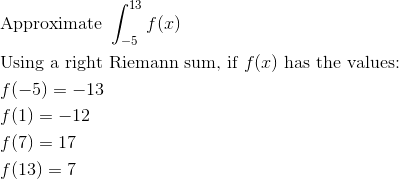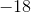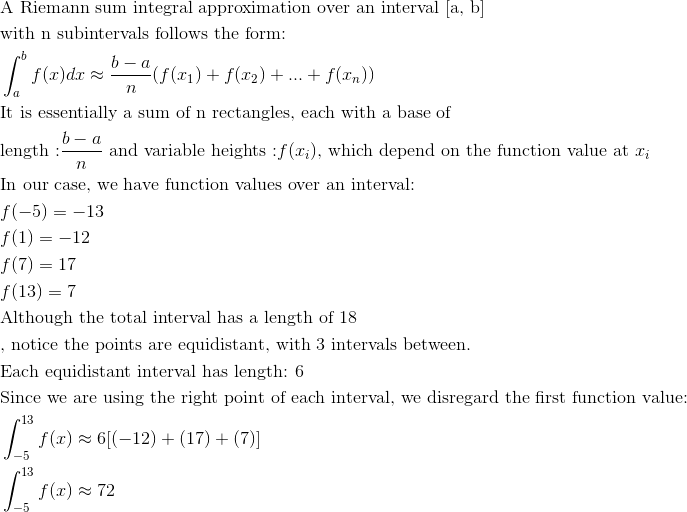# AP Calculus AB : Riemann sums (left, right, and midpoint evaluation points)

## Example Questions

← Previous 1 3

### Example Question #1 : Riemann Sums (Left, Right, And Midpoint Evaluation Points)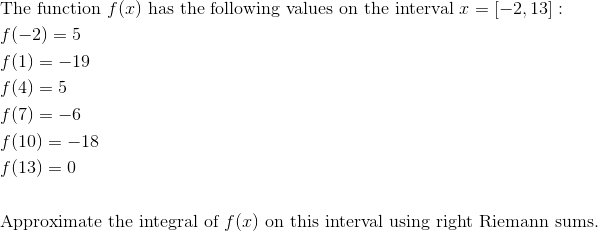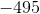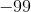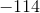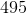Explanation: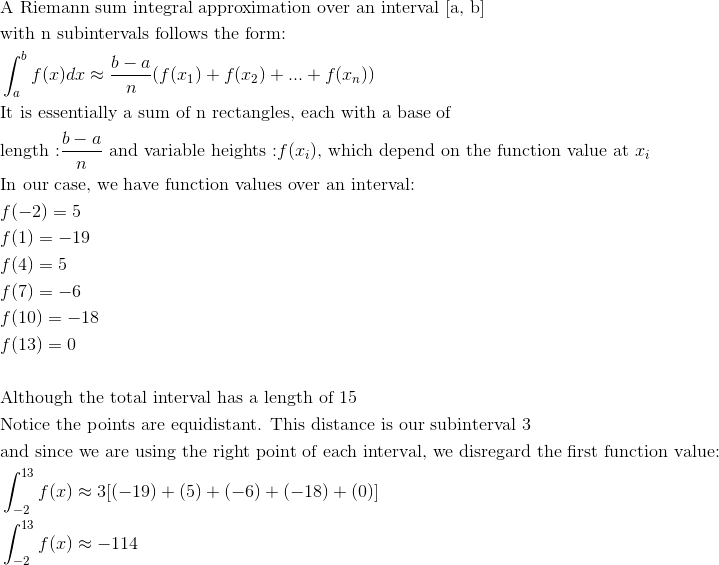### Example Question #2 : Riemann Sums (Left, Right, And Midpoint Evaluation Points)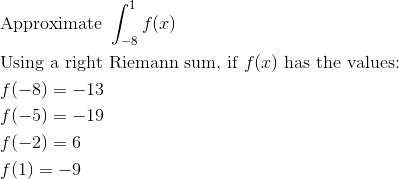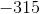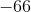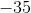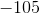Explanation: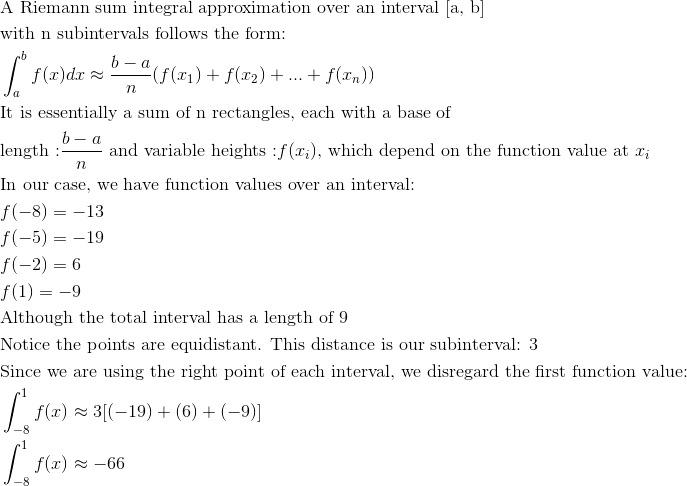### Example Question #3 : Riemann Sums (Left, Right, And Midpoint Evaluation Points)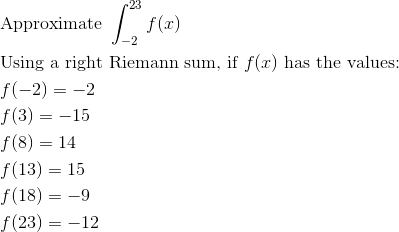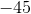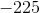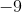Explanation: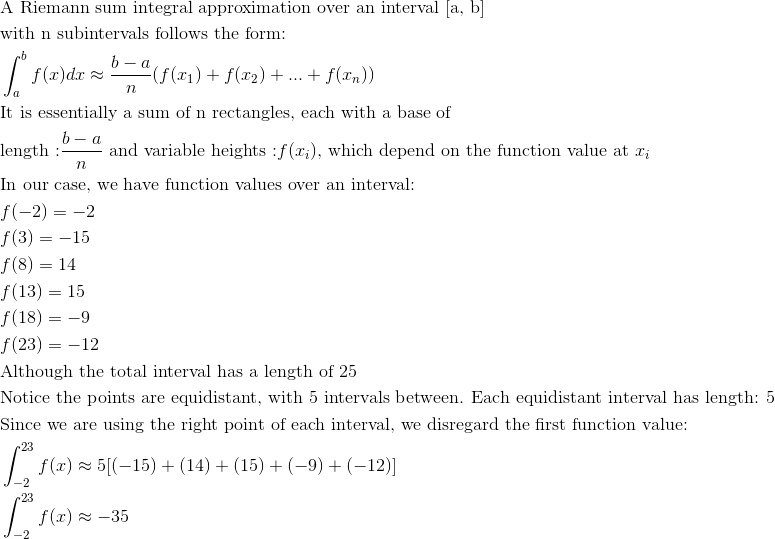### Example Question #4 : Riemann Sums (Left, Right, And Midpoint Evaluation Points)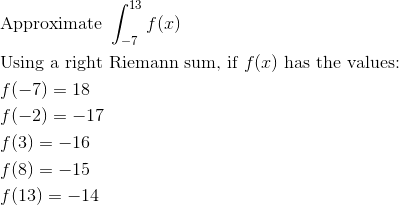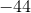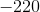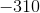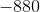Explanation: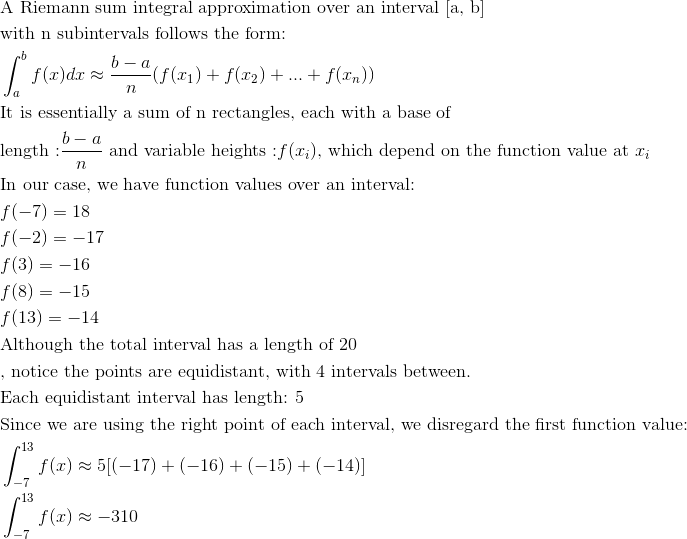### Example Question #5 : Riemann Sums (Left, Right, And Midpoint Evaluation Points)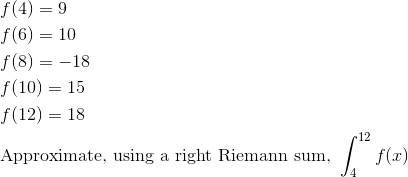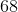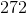Explanation: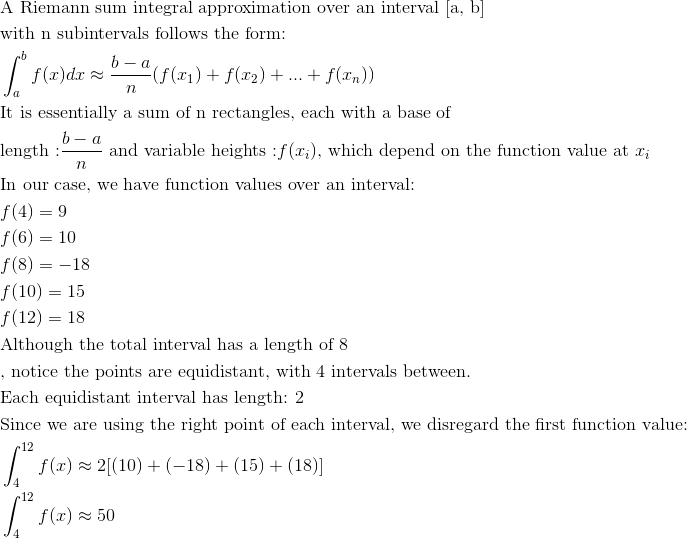### Example Question #6 : Riemann Sums (Left, Right, And Midpoint Evaluation Points)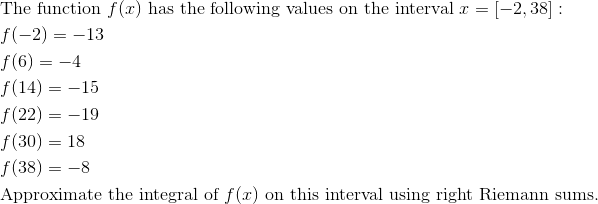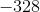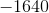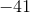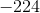Explanation: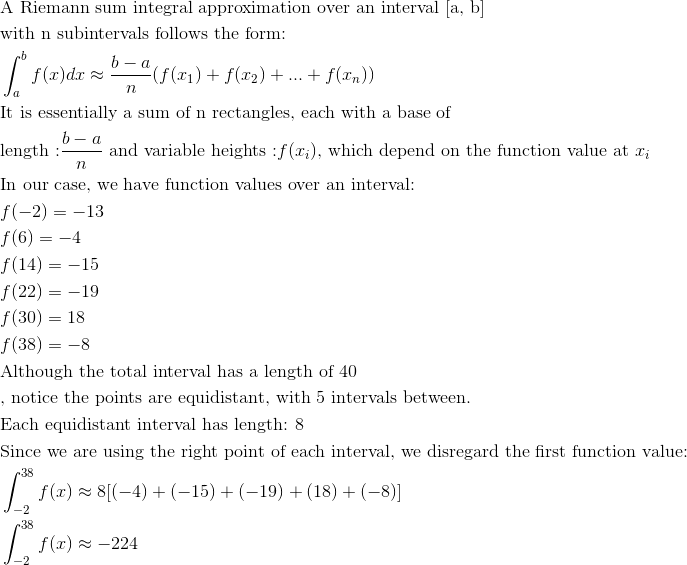### Example Question #7 : Riemann Sums (Left, Right, And Midpoint Evaluation Points)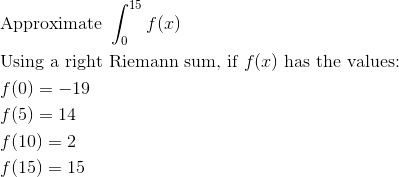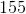Explanation: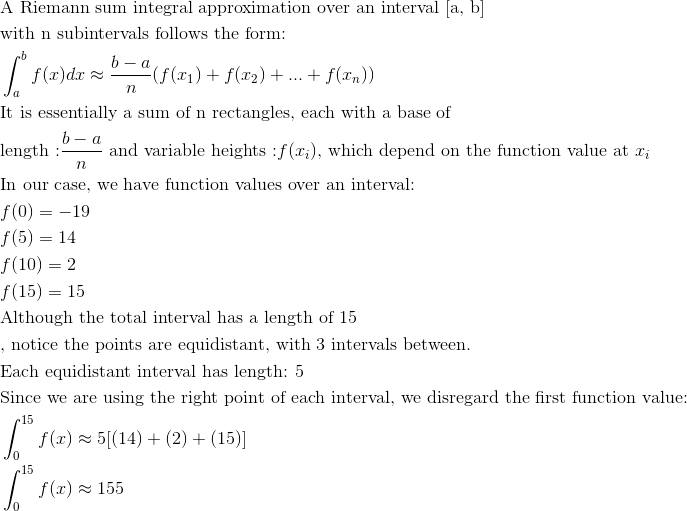### Example Question #8 : Riemann Sums (Left, Right, And Midpoint Evaluation Points)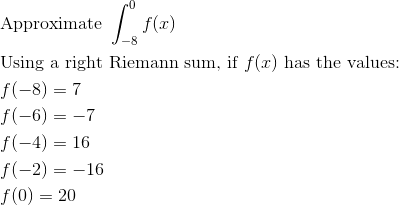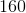Explanation: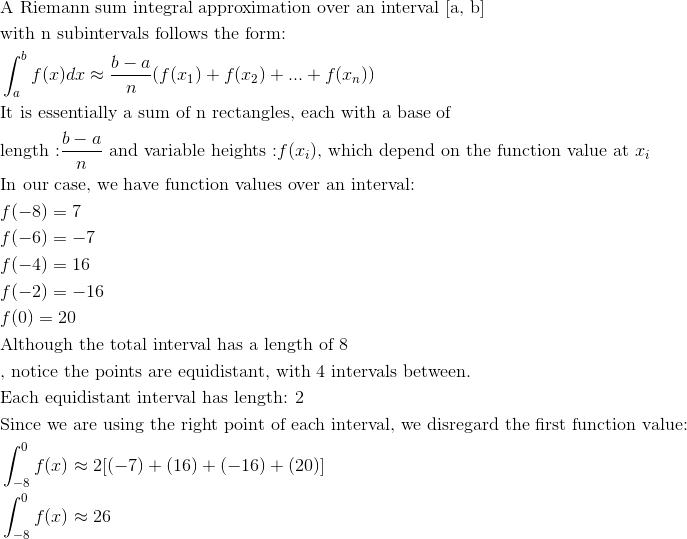### Example Question #9 : Riemann Sums (Left, Right, And Midpoint Evaluation Points)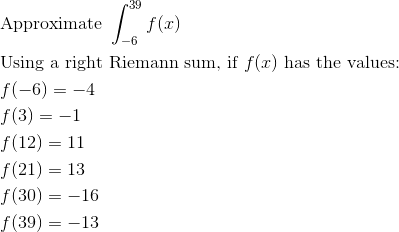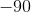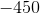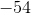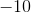Explanation: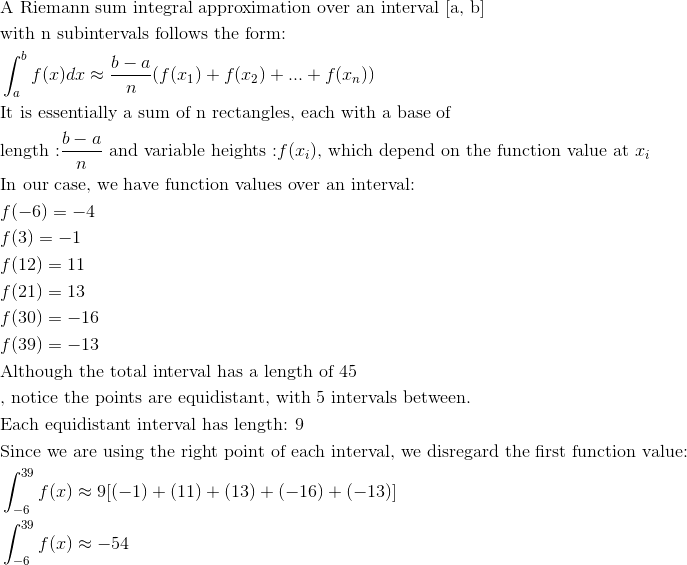### Example Question #10 : Riemann Sums (Left, Right, And Midpoint Evaluation Points)